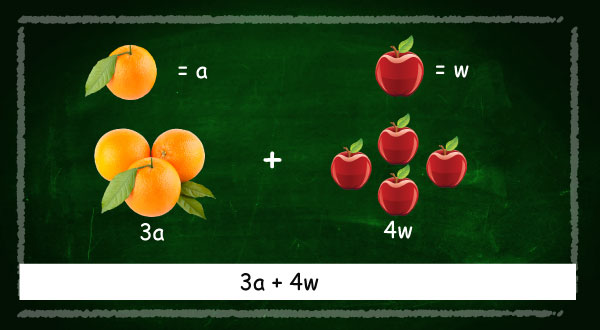Simplifying algebraic equations

Mathematics dealing with numbers and letters is called Algebra.

Algebra is mathematics intended to help explain certain types of problems quicker and easier.

e.gWanja’s age is four times the age of Wanjiku.

In algebra we use letters, symbols and numbers to solve problems (puzzles).

Earlier in class one we solved mathematic problems like;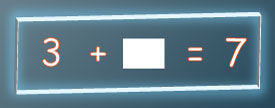What is the missing number?

In algebra instead of blank boxes we use letters like “b” , “x”,  “y

So in the above puzzle we would write;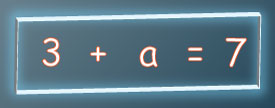The letter means “we do not know” therefore called the “unknown

Lets solve this puzzle.We used a letter because its easier to write letters and if we have several unknowns then we can use different letters instead of keeping on drawing shapes.

In algebraic equations we refer to the letter as an unknown, and the numbers a constant.

And,Is an equation!

#### Simplifying algebraic expressions & Use of brackets in algebraic expressions.

Simplify means to make simpler or easier to use format.

The algebraic expression in the “simple form”.

#### For example

Simplify 3x +4x- 2

Requires that we combine like terms to get; 7x-2 (which is easier to use)

Another example,

Simplify; 3x (4x-2)

Requires we remove the brackets first by multiplying through to get;

12x-6x=6x (which is easier to use)

## Simplify means to make simpler or easier to use format.

The algebraic expression in the “simple form”.

For example

Simplify; 20 (x +x- 2)

Requires that we combine like terms to get; 40x-40(which is easier to use)

#### Example 1

Simplify  6(2x - 3) + 4(x + 2)

step one: open the brackets by multiplying through

step two: combine the like terms (remember to carry the plus or minus sign)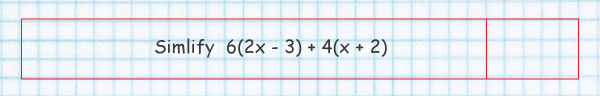#### Solution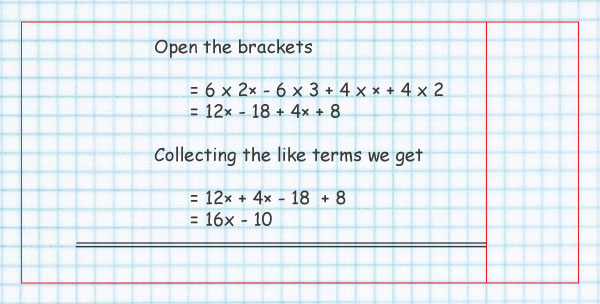#### Example 2

simplify 4y-5y + 8y-2y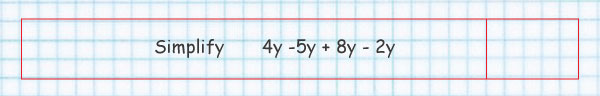And here is the solution

Please study it careful to learn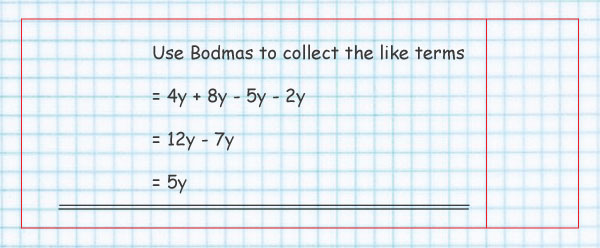We add or subtract like terms

#### Look at the image below: